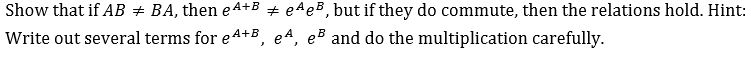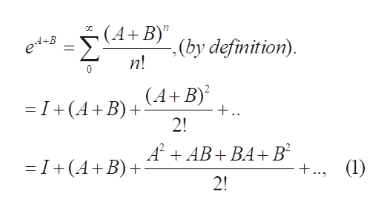# Show that if ABBA, then eA+BeAe, but if they do commute, then the relations hold. Hint:Write out several terms for e4+B, e4, e and do the multiplication carefully

Question
64 viewshelp_outlineImage TranscriptioncloseShow that if AB BA, then eA+BeAe, but if they do commute, then the relations hold. Hint: Write out several terms for e4+B, e4, e and do the multiplication carefully fullscreen
check_circle

Step 1

The problem concerns the commutativity properties of the exponentials of square matrices

Step 2

Definition of e^X= exp(X), X a square matrix

Step 3

Consider e^(A+B) and e^A times...help_outlineImage Transcriptionclose(A+B) (by definition). 4+B n! =I+ (A+B)+ (4+ B)? 2! +.. AAB+BA+B =I+(A+B) +., (1) 2! fullscreen

### Want to see the full answer?

See Solution

#### Want to see this answer and more?

Solutions are written by subject experts who are available 24/7. Questions are typically answered within 1 hour.*

See Solution
*Response times may vary by subject and question.
Tagged in

### Math